## 1. 线性回归的交叉验证

${ min_{\pmb{x}} ||\pmb{A} \pmb{x}- \pmb{b}||^2 }$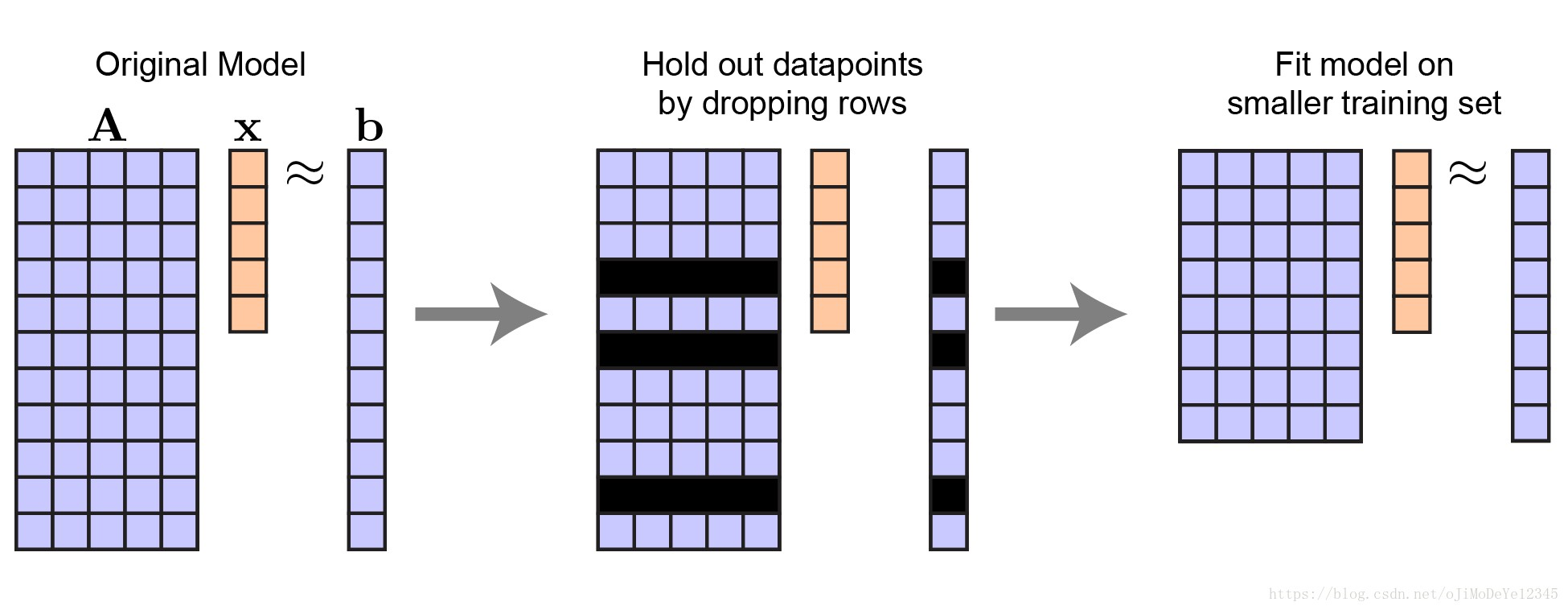Figure 1. 线性回归中留出数据样本的过程. 向量 ${ \pmb{x} }$ 的长度保持不变.

${ \hat{\pmb{x}} = argmin_{\pmb{x}} || A_{tr} \pmb{x}- \pmb{b}_{tr} ||^2 }$

## 2. PCA 的交叉验证

PCA 分解可以看做优化问题：

${ mini_{\pmb{U}, \pmb{V}} || \pmb{U}\pmb{V}^T- \pmb{Y} ||^2 }$

Everything you did and didn't know about PCA

[Dimensionality Reduction for Matrix- and Tensor-Coded Data [Part 1]](https://cbmm.mit.edu/video/dimensionality-reduction-matrix-and-tensor-coded-data-part-1)

Generalized Low Rank Models - Book

PCA 分解图解为：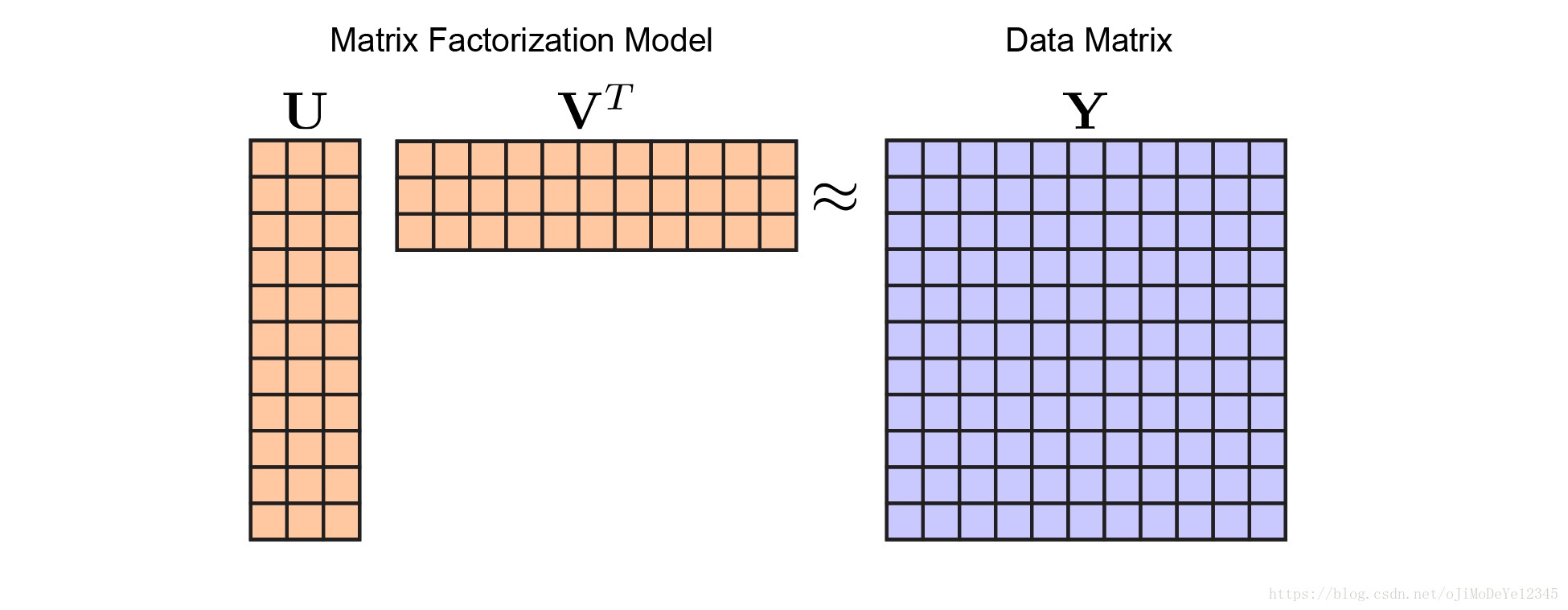Figure 2. 矩阵分解模型. 不同颜色表示不同的模型参数，矩阵${ \pmb{U} }$ 和 ${ \pmb{V} }$ 为橙色，数据 ${ \pmb{Y} }$ 为蓝色. 通过优化矩阵 ${ \pmb{U} }$ 和 ${ \pmb{V} }$ 来最小化关于 ${ \pmb{Y} }$ 的重构误差.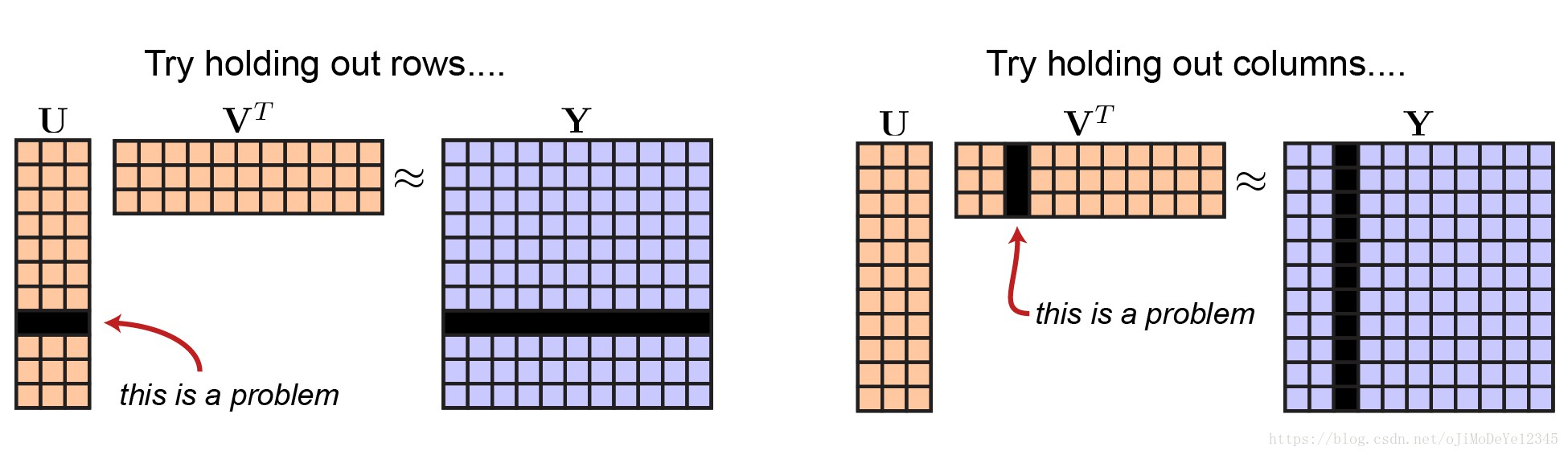Figure 3. 交叉验证矩阵分解的不完整方案

 - 留出矩阵 ${ \pmb{Y} }$ 的一些行，然后在训练集上对矩阵 ${ \pmb{U} }$ 的行的子集，来拟合矩阵 ${ \pmb{V} }$.
 - 估计矩阵 ${ \pmb{V} }$ 的表现，稍微改变下交叉验证规则，采用测试数据(即留出的行) 来对矩阵 ${ \pmb{U} }$ 估计.

Cross-validation of component models: A critical look at current methods

Bi-cross-validation of the SVD and the nonnegative matrix factorization

## 3. PCA 交叉验证的原因

${ min_x ||\pmb{A} \pmb{x}- \pmb{b} ||^2 + \lambda ||\pmb{x}||_1 }$Figure 4. LASSO 的交叉验证图.0 黑色虚线表示最佳的 ${ \lambda }$ 值选择，其能够在留出测试数据集上得到最小的误差.

PCA 还有另一个重要的参数：模型主成分(components)个数.

Automatic choice of dimensionality for PCA

The Optimal Hard Threshold for Singular Values is 4/sqrt(3)

Model averaging and dimension selection for the singular value decomposition

Determining the number of clusters in a data set

## 4. 矩阵分解的交叉验证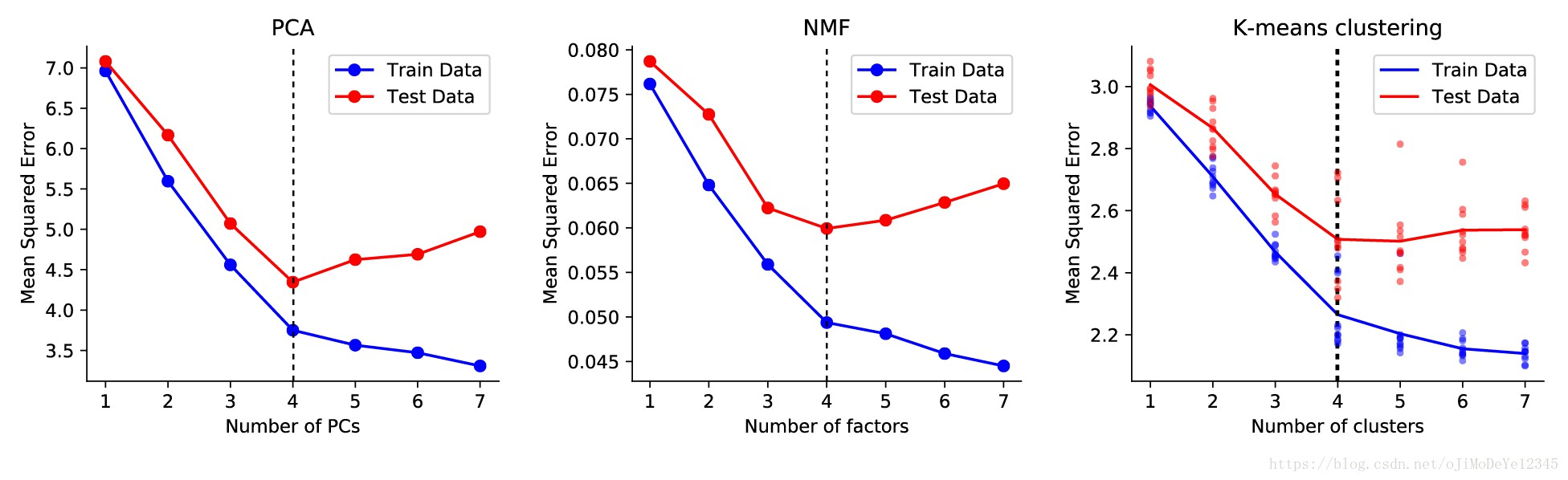Figure 5. 一些矩阵分解模型的交叉验证图示. 其中，K-means聚类的实现有一点噪声，故进行了多次优化的平均.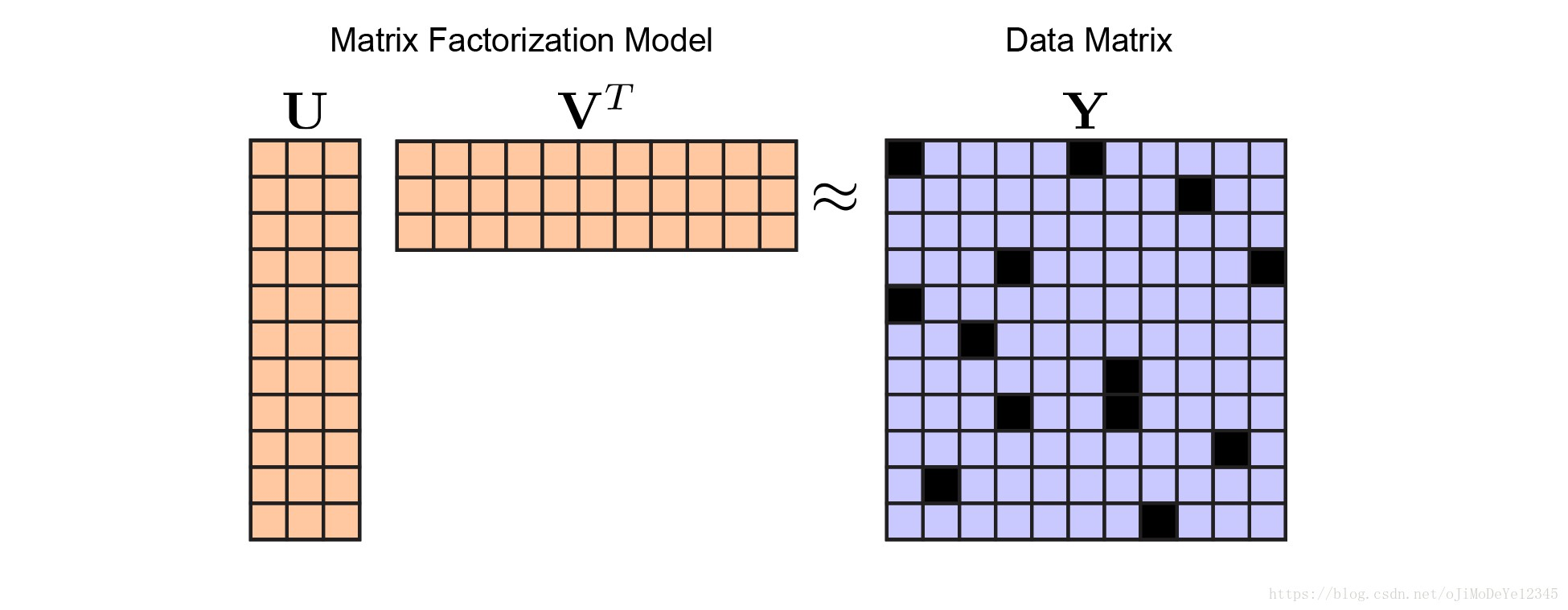Figure 6. 交叉验证较好的解决方案是随机的留出数据. 重要的是，只要数据矩阵 ${ \pmb{Y} }$ 的中的某一行或某一列没有被完全丢失，则仍然可以学习矩阵 ${ \pmb{U} }$ 和 ${ \pmb{V} }$ 的所有参数. 直观上来说，仍应保持 ${ \pmb{Y} }$ 中每一行或每一列至少有 ${ r }$ 个值.

${ min_{\pmb{U}, \pmb{V}} ||\pmb{M} \circ (\pmb{U} \pmb{V}^T- \pmb{Y})||_F^2 }$ （3）

${ ||(1- \pmb{M}) \circ (\pmb{U} \pmb{V}^T- \pmb{Y})||_F^2 }$

${ min_{\hat{\pmb{Y}}} ||\hat{\pmb{Y}}||_* }$

${ s.t. \pmb{M} \circ \hat{\pmb{Y}} = \pmb{M} \circ \pmb{Y} }$

Everything you did and didn't know about PCA

Dimensionality reduction matrix and tensor coded data part1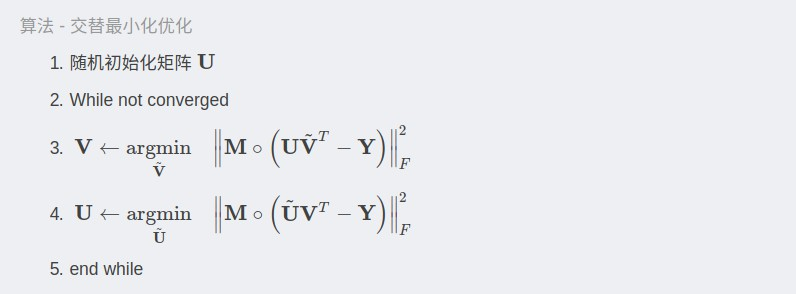Understanding Alternating Minimization for Matrix Completion 中采用交替最小化来求解矩阵补全问题，但其实现过程仍未完成.

### 4.1 PCA 实现

def censored_lstsq(A, B, M):
"""
Least squares of M * (AX - B) over X, where M is a masking matrix.
"""
rhs = np.dot(A.T, M * B).T[:,:,None] # n x r x 1 tensor
T = np.matmul(A.T[None,:,:], M.T[:,:,None] * A[None,:,:])
return np.linalg.solve(T, rhs).reshape(A.shape, B.shape)

def cv_pca(data, rank, p_holdout=.1):
"""
Fit PCA while holding out a fraction of the dataset.
"""
M = np.random.rand(data.shape)

# fit pca
U = np.random.randn(data.shape, rank)
for itr in range(20):
Vt = censored_lstsq(U, data, M)
U = censored_lstsq(Vt.T, data.T, M.T).T

# We could orthogonalize U and Vt and then rotate to align
# with directions of maximal variance, but we won't bother.

# return result and test/train error
resid = np.dot(U, Vt) - data
train_err = np.mean(resid[M]**2)
test_err = np.mean(resid[~M]**2)
return train_err, test_err

 - 这里简洁起见，没有检查收敛性. 在实际代码中，需要监视重构误差或者 ${ \pmb{U} }$ 和 ${ \pmb{V}^T }$ 的变化情况，当收敛时结束循环.
 - censored_1stsq 函数的实现不是最优的. Solving Least-Squares Regression with Missing Data
 - 如果数据缺失过多，如一整行或一整列的数据被留出，则由于其是奇异矩阵/不可逆矩阵(singular/non-invertible matrix)，censored——1stsq函数会计算失败.

### 4.2 NMF 和 K-means 实现

nonnegfac-python 详细实现了非负最小二乘.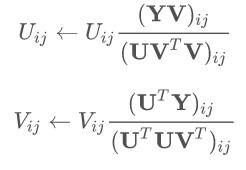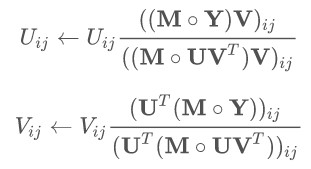K-means 聚类稍微复杂一点，但基本的交叉验证思想是相同的. 可参见：

## 5. 总结Figure 7. 交叉验证数据留出方法. From Bi-cross-validation of the SVD and the nonnegative matrix factorization

Cross-Validation for Unsupervised Learning - K-means 聚类，PhD 论文

Cross-validation of component models: A critical look at current methods

Last modification：April 28th, 2021 at 10:43 am